# Digital Image Procesing Unitary Transforms Discrete Fourier Trasform

• Slides: 48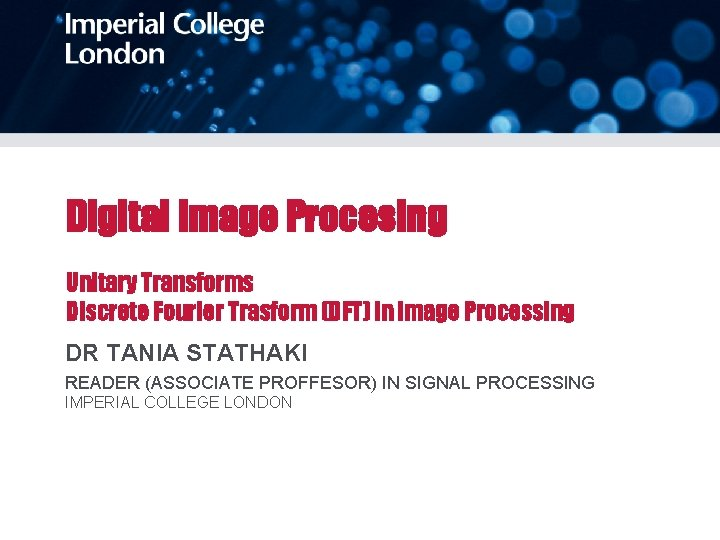Digital Image Procesing Unitary Transforms Discrete Fourier Trasform (DFT) in Image Processing DR TANIA STATHAKI READER (ASSOCIATE PROFFESOR) IN SIGNAL PROCESSING IMPERIAL COLLEGE LONDON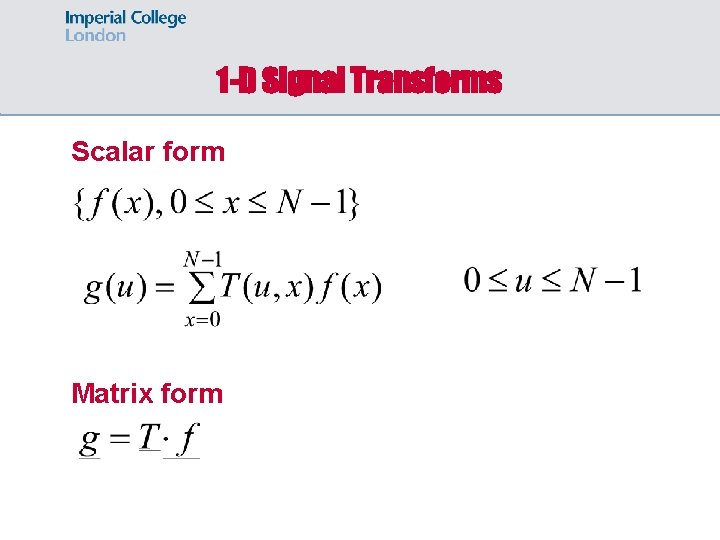1 -D Signal Transforms Scalar form Matrix form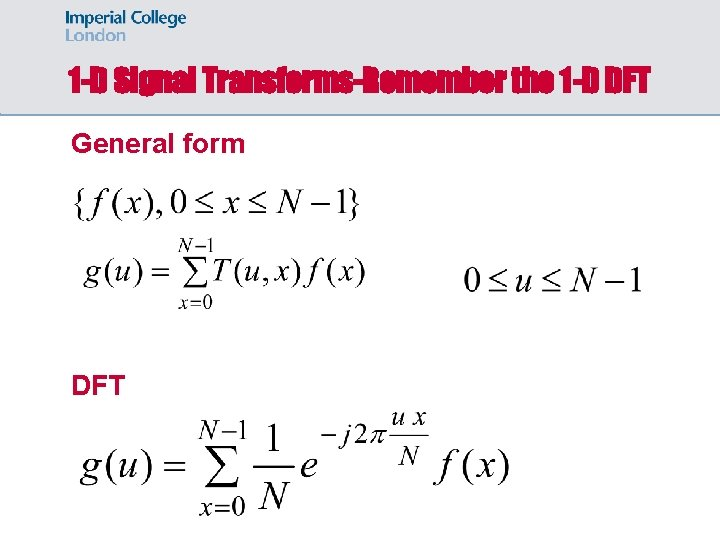1 -D Signal Transforms-Remember the 1 -D DFT General form DFT1 -D Inverse Signal Transforms-General Form Scalar form Matrix form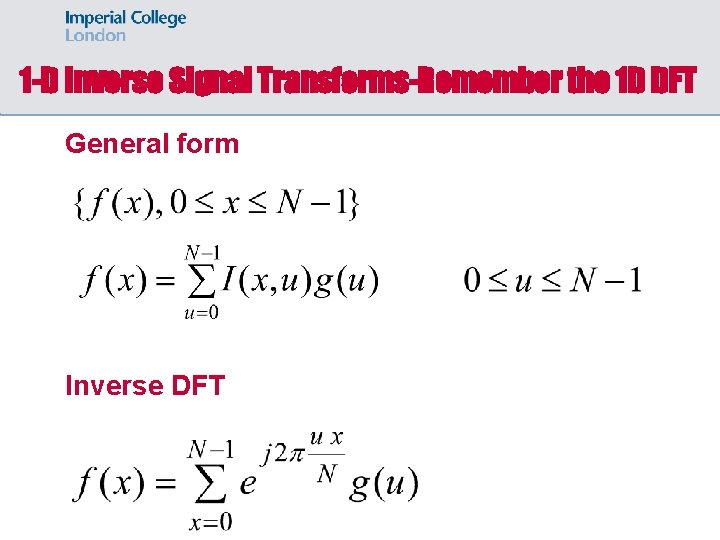1 -D Inverse Signal Transforms-Remember the 1 D DFT General form Inverse DFT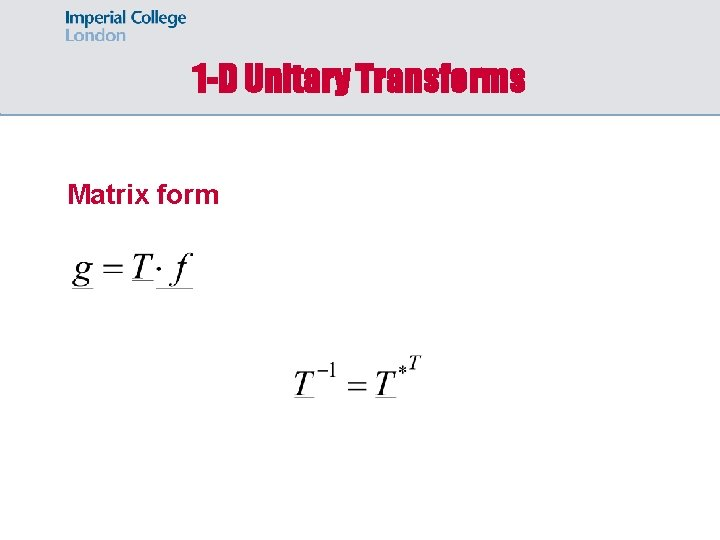1 -D Unitary Transforms Matrix formSignal ReconstructionWhy do we use Image Transforms? Often, image processing tasks are best performed in a domain other than the spatial domain. Key steps: • Transform the image • Carry the task(s) of interest in the transformed domain. • Apply inverse transform to return to the spatial domain.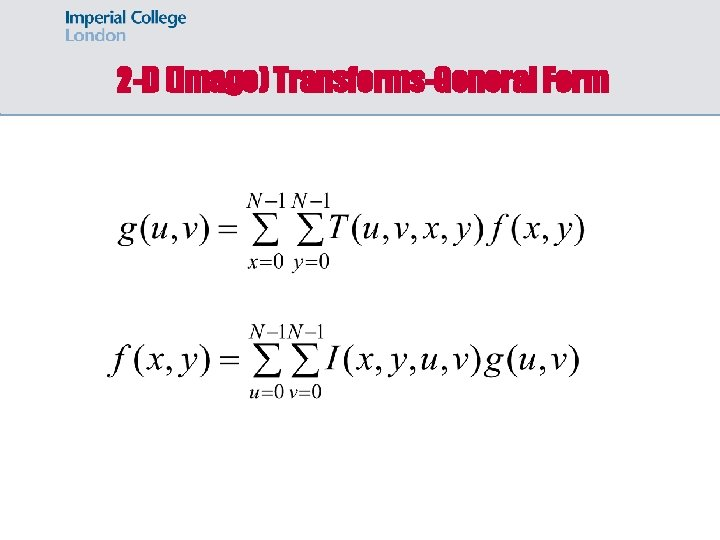2 -D (Image) Transforms-General Form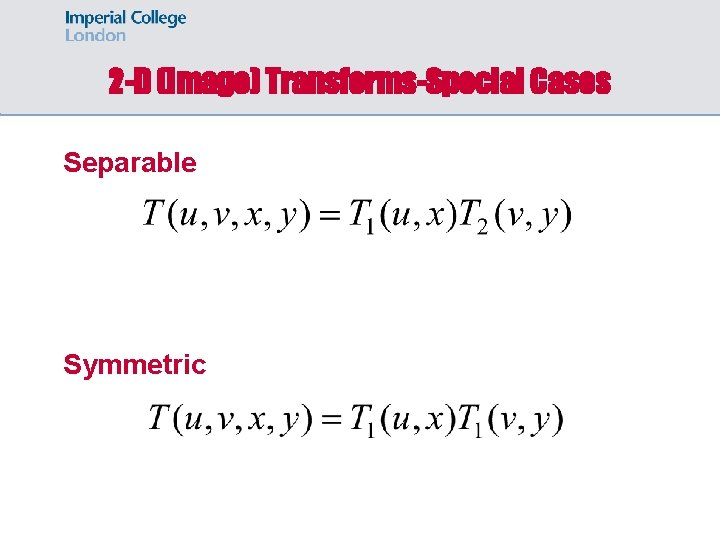2 -D (Image) Transforms-Special Cases Separable Symmetric2 -D (Image) Transforms-Special Cases (cont. ) Separable and Symmetric Separable, Symmetric and UnitaryEnergy Preservation 1 -D 2 -D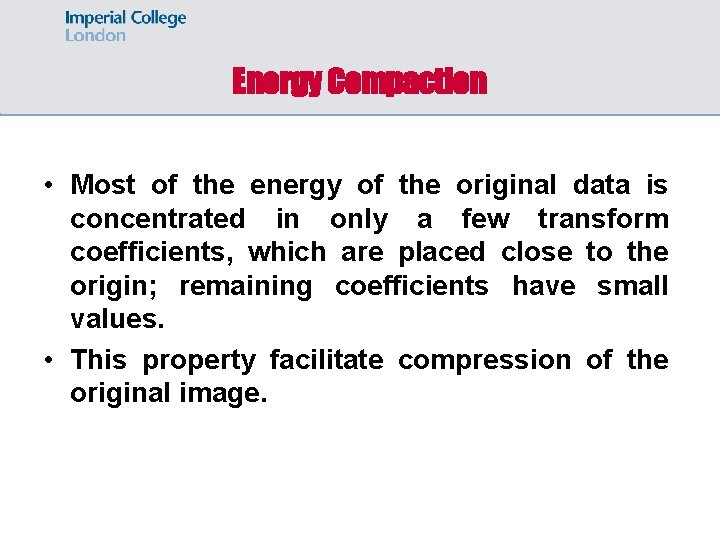Energy Compaction • Most of the energy of the original data is concentrated in only a few transform coefficients, which are placed close to the origin; remaining coefficients have small values. • This property facilitate compression of the original image.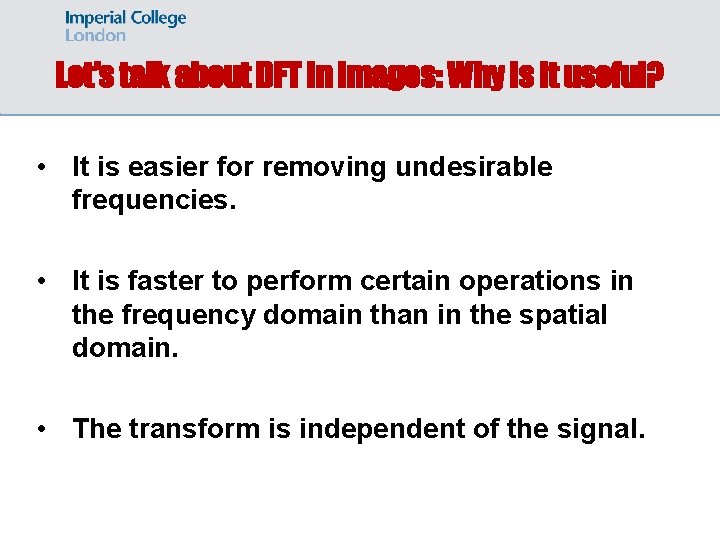Let’s talk about DFT in images: Why is it useful? • It is easier for removing undesirable frequencies. • It is faster to perform certain operations in the frequency domain than in the spatial domain. • The transform is independent of the signal.Example: Removing undesirable frequencies Example of removing a high frequency using the transform domain noisy signal frequencies Result after removing high frequencies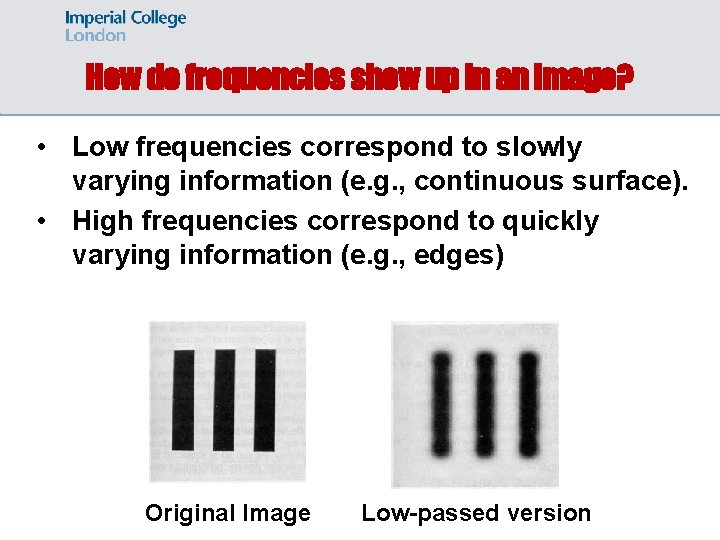How do frequencies show up in an image? • Low frequencies correspond to slowly varying information (e. g. , continuous surface). • High frequencies correspond to quickly varying information (e. g. , edges) Original Image Low-passed version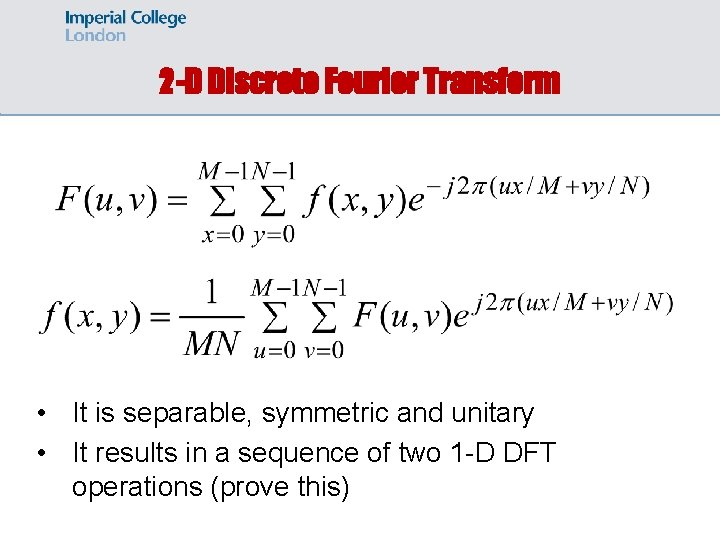2 -D Discrete Fourier Transform • It is separable, symmetric and unitary • It results in a sequence of two 1 -D DFT operations (prove this)Visualizing DFT • • • The dynamic range of F(u, v) is typically very large Small values are not distinguishable We apply a logarithmic transformation to enhance small values. original image before transformation after transformation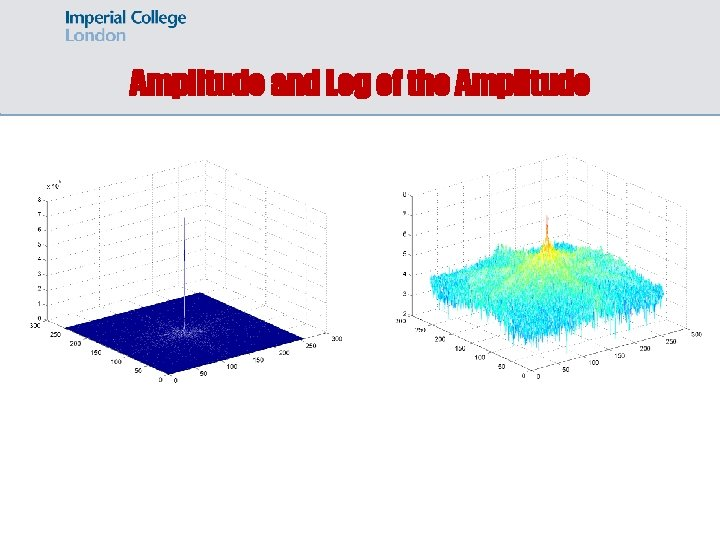Amplitude and Log of the AmplitudeAmplitude and Log of the Amplitude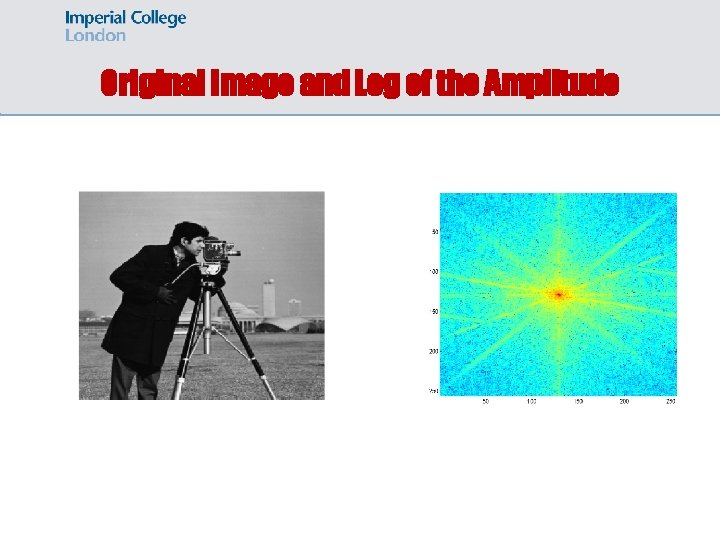Original Image and Log of the Amplitude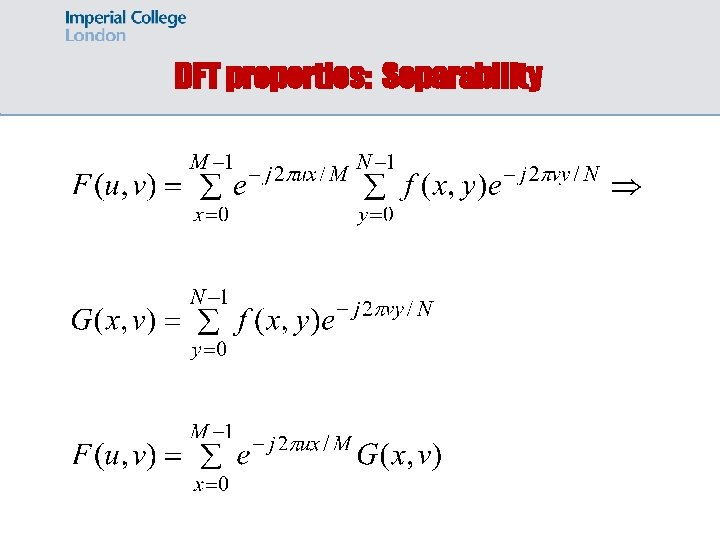DFT properties: Separability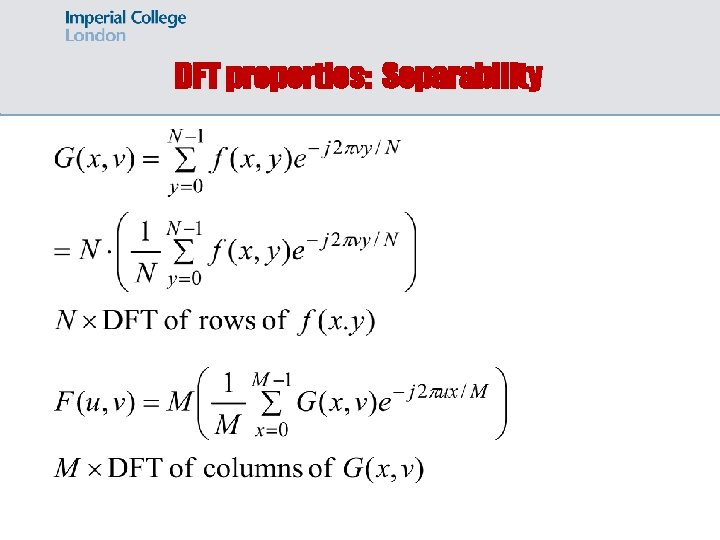DFT properties: Separability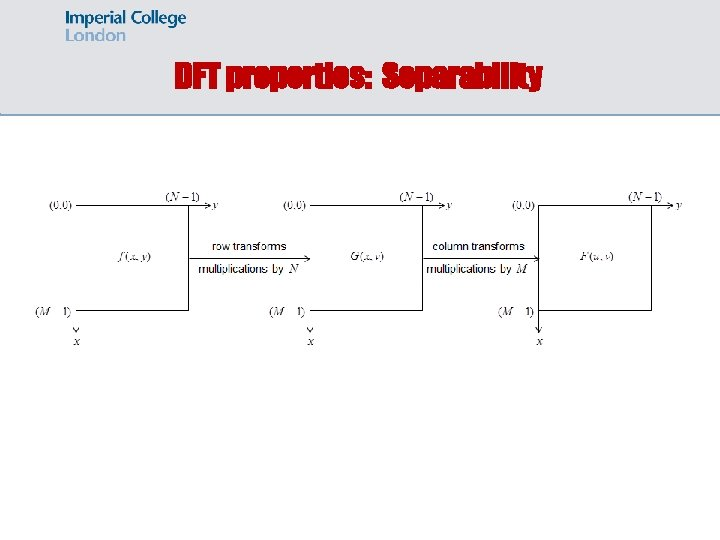DFT properties: SeparabilityDFT properties: Separability The DFT and its inverse are periodic.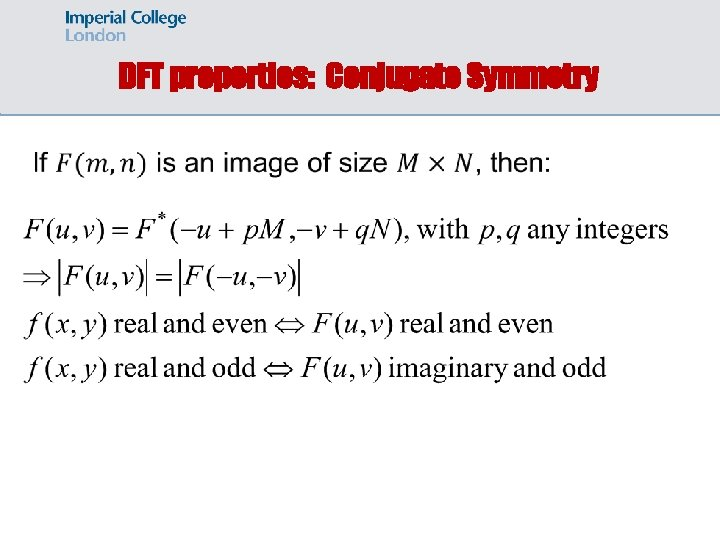DFT properties: Conjugate Symmetry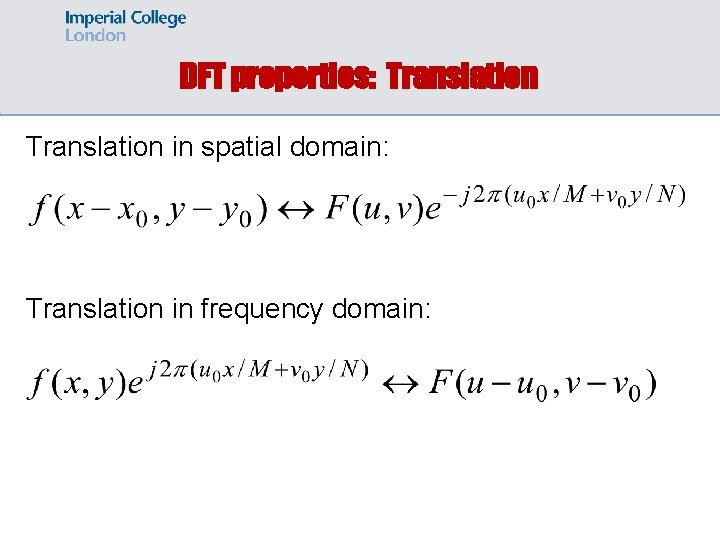DFT properties: Translation in spatial domain: Translation in frequency domain:DFT properties: Translation Warning: to show a full period, we need to translate the origin of the transform at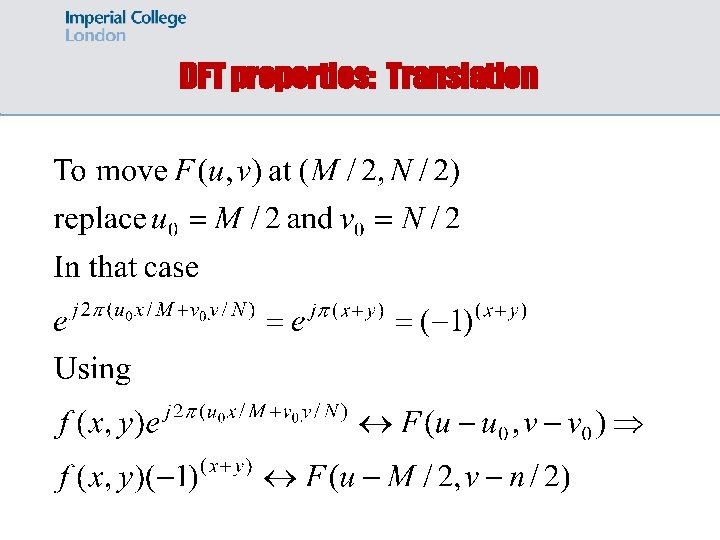DFT properties: Translation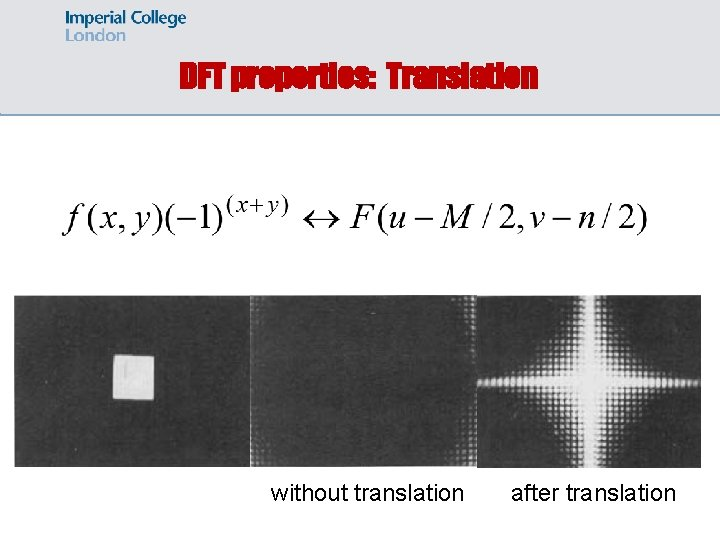DFT properties: Translation without translation after translation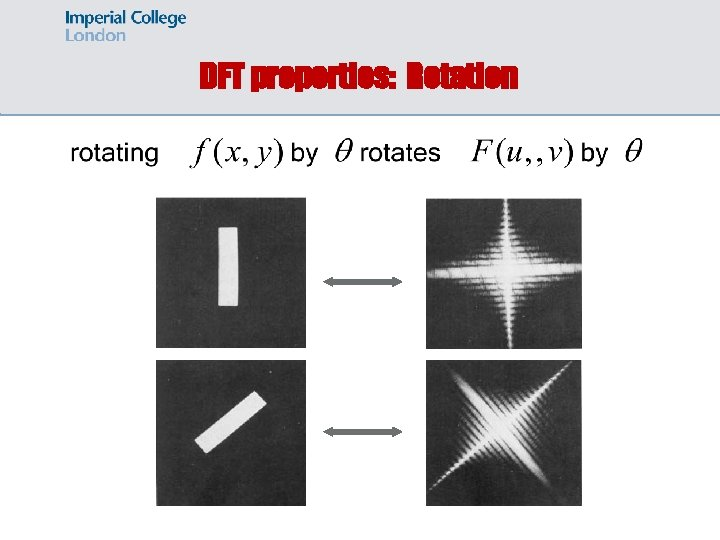DFT properties: Rotation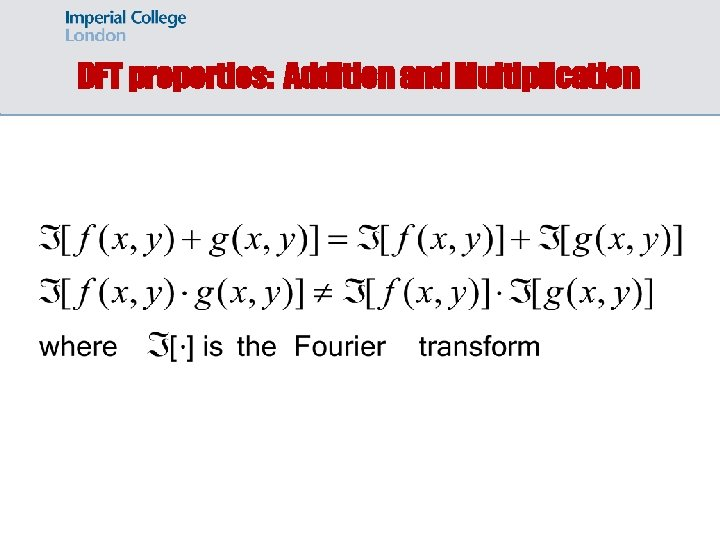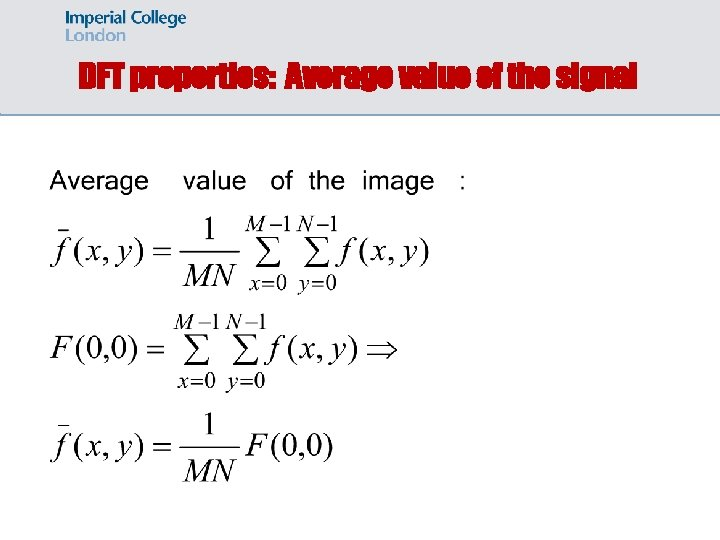DFT properties: Average value of the signal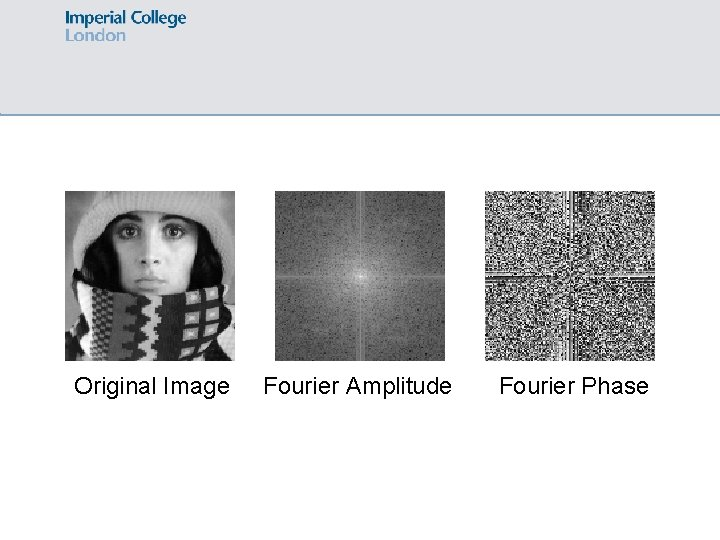Original Image Fourier Amplitude Fourier Phase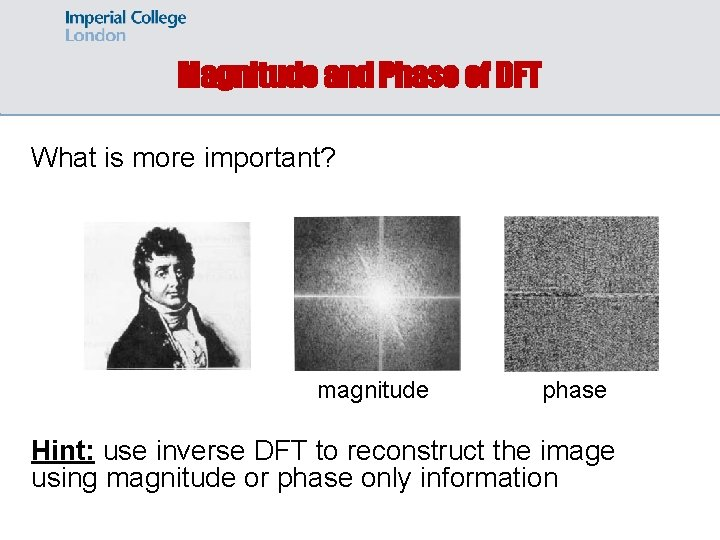Magnitude and Phase of DFT What is more important? magnitude phase Hint: use inverse DFT to reconstruct the image using magnitude or phase only information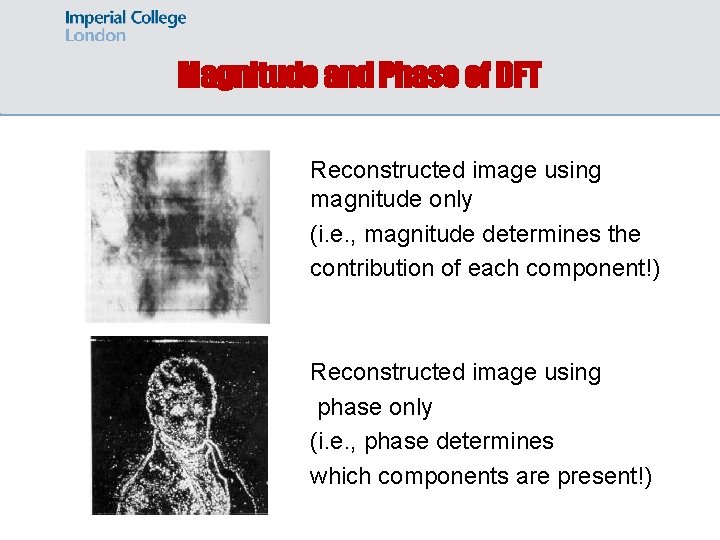Magnitude and Phase of DFT Reconstructed image using magnitude only (i. e. , magnitude determines the contribution of each component!) Reconstructed image using phase only (i. e. , phase determines which components are present!)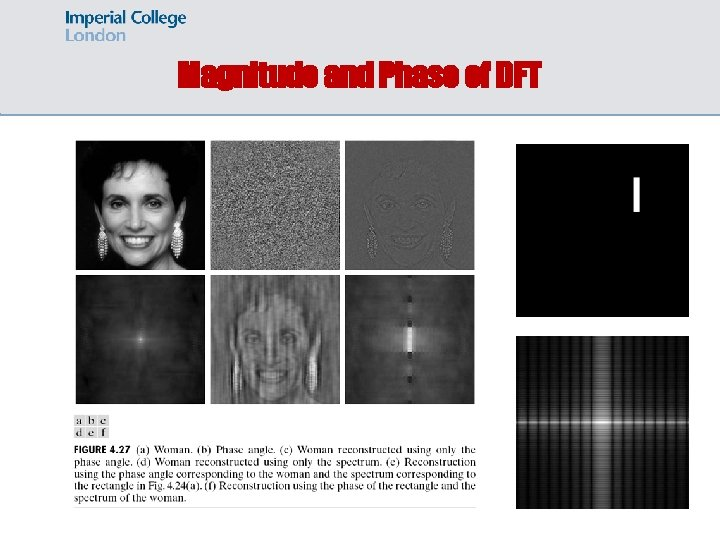Magnitude and Phase of DFTLow pass filtering using DFT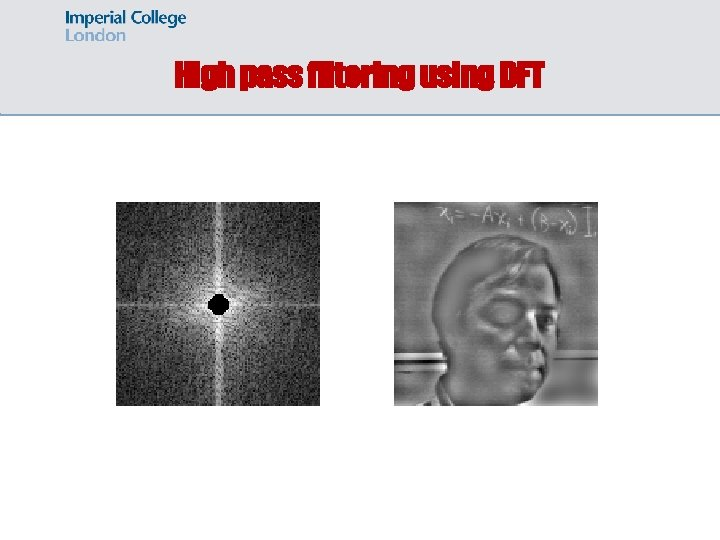High pass filtering using DFT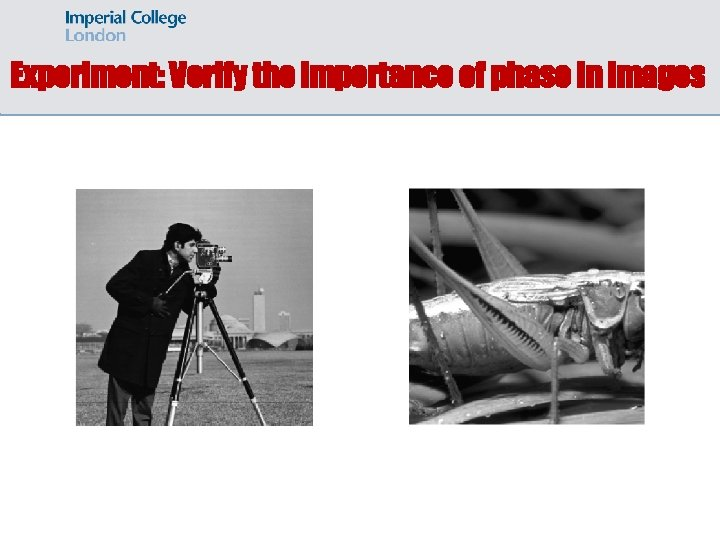Experiment: Verify the importance of phase in images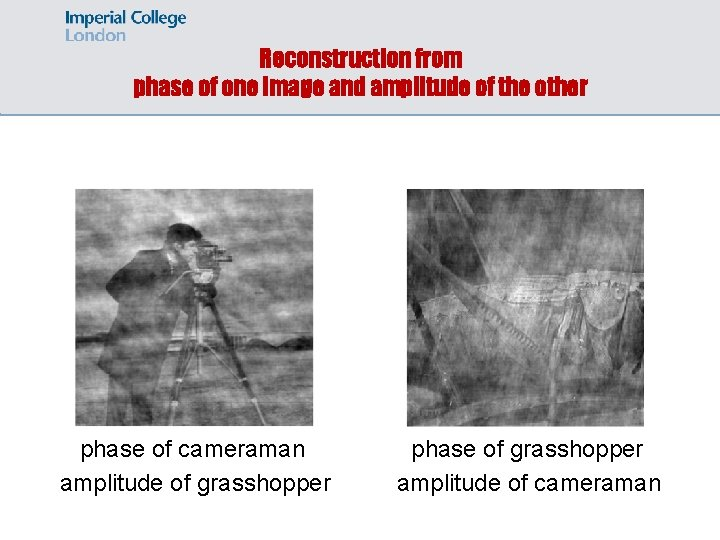Reconstruction from phase of one image and amplitude of the other phase of cameraman amplitude of grasshopper phase of grasshopper amplitude of cameramanExperiment: Verify the importance of phase in imagesReconstruction from phase of one image and amplitude of the other phase of buffalo amplitude of rocks phase of rocks amplitude of buffalo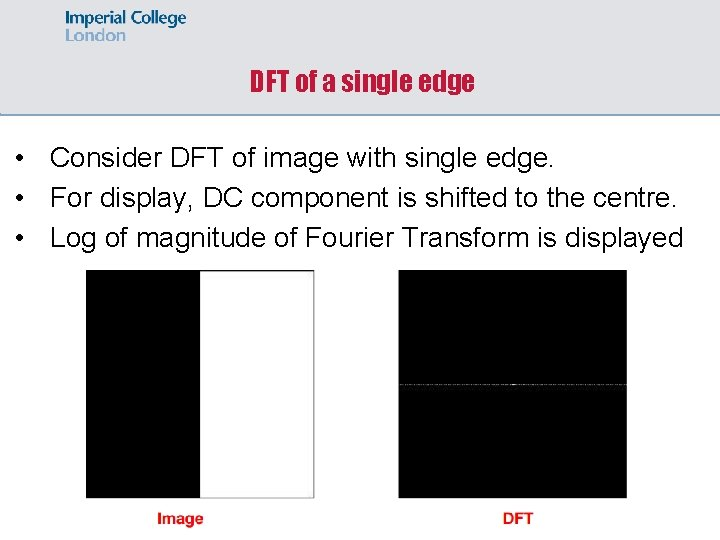DFT of a single edge • Consider DFT of image with single edge. • For display, DC component is shifted to the centre. • Log of magnitude of Fourier Transform is displayedDFT of a box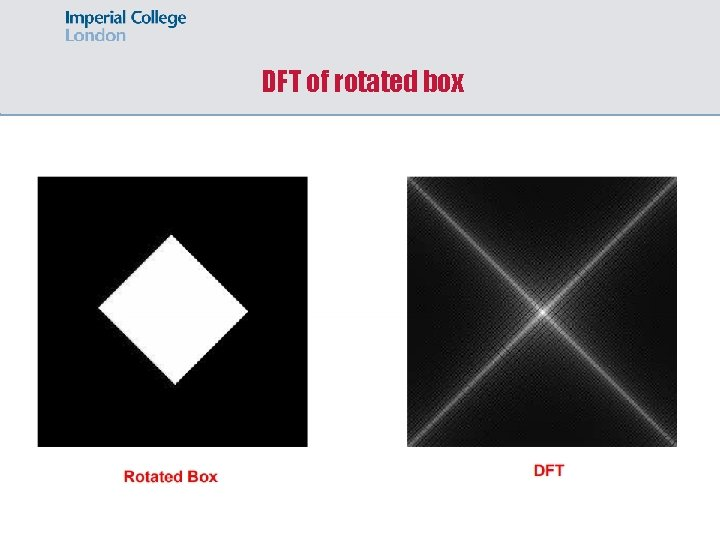DFT of rotated box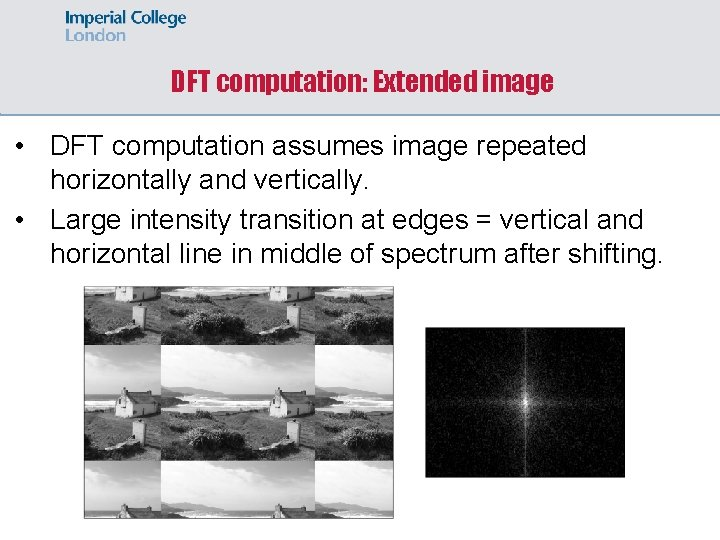DFT computation: Extended image • DFT computation assumes image repeated horizontally and vertically. • Large intensity transition at edges = vertical and horizontal line in middle of spectrum after shifting.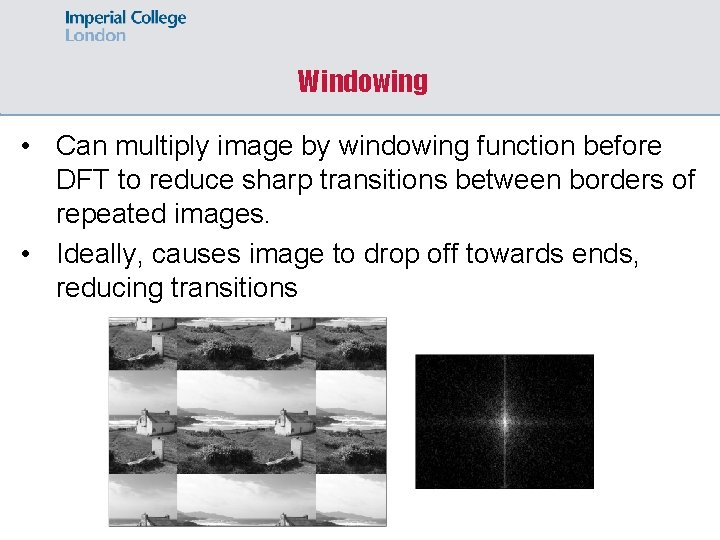Windowing • Can multiply image by windowing function before DFT to reduce sharp transitions between borders of repeated images. • Ideally, causes image to drop off towards ends, reducing transitions